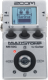# High gain sound of MS 1959 with CentaGold boosting

Discussion in 'Zoom MS-50G' started by m-shim, May 15, 2022.

1. High gain sound of MS 1959 with CentaGold boostingDevice: Zoom Ms-50g
Firmware: 3.10

Name on device: MS1959HG
Optimized for: Phones/Speaker

Effects chain:High gain sound of Marshall 1959 Plexi boosted by CentaGold with Spring reverb.

Effect: "ZNR" (Dynamics / Filter), active - "yes"
"THRSH" = 13
"DETCT" = GtrIn
"Level" = 100

Effect: "ParaEQ" (Dynamics / Filter), active - "yes"
"Freq1" = 5.0kHz
"Q1" = 2
"Gain1" = 5
"Freq2" = 630Hz
"Q2" = 1
"Gain2" = 5
"Level" = 100

Effect: "CentaGold" (Overdrive / Distortion), active - "yes"
"GAIN" = 30
"TRBL" = 70
"OUT" = 22

Effect: "MS 1959" (Amp simulator), active - "yes"
"Gain" = 60
"Tube" = 65
"Level" = 25
"Trebl" = 70
"Middl" = 70
"Bass" = 55
"Prese" = 65
"CAB" = MS 1959 4x12
"OUT" = LINE

Effect: "Delay" (Delay / Reverb), active - "yes"
"Time" = 325
"F.B" = 15
"Mix" = 25
"HiDMP" = 10
"P-P" = MONO
"Level" = 100
"Tail" = On

Effect: "Spring" (Delay / Reverb), active - "yes"
"Decay" = 15
"Tone" = 8
"Mix" = 40
"PreD" = 20
"Level" = 100
"Tail" = On

Note: This is a patch file, you will need to download and install the ToneLib-Zoom software to use the patch.

File size:
494 bytes
Views:
162